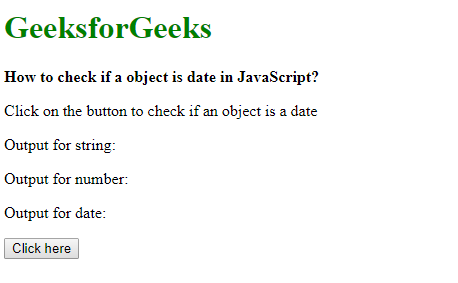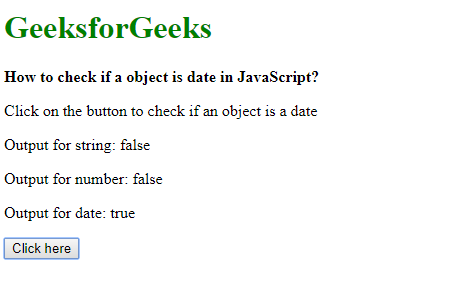# How to check whether an object is a date?

Method 1: Using instanceof operator: The instanceof operator checks whether the prototype property of a constructor appears anywhere in the prototype chain of an object. In this case, it is used to check whether the object is an instance of the Date object or not. A true value means that it does match the object specified.
The validity of the date in the Date object can be checked with the !isNan() function. It returns true if the date is not invalid.

Syntax:

`object instanceof Date`

Example:

 ` ` `<``html``> ` ` `  `<``head``> ` `    ``<``title``> ` `        ``How to check an object is ` `        ``date in JavaScript? ` `    `` ` ` ` ` `  `<``body``> ` `    ``<``h1` `style``=``"color: green"``> ` `        ``GeeksforGeeks ` `    `` ` `     `  `    ``<``b``> ` `        ``How to check if a object is ` `        ``date in JavaScript? ` `    `` ` `     `  `    ``<``p``> ` `        ``Click on the button to check ` `        ``if an object is a date ` `    `` ` `     `  `    ``<``p``> ` `        ``Output for string:  ` `        ``<``span` `class``=``"outputString"``> ` `    `` ` `     `  `    ``<``p``> ` `        ``Output for number:  ` `        ``<``span` `class``=``"outputNumber"``> ` `    `` ` `     `  `    ``<``p``> ` `        ``Output for date:  ` `        ``<``span` `class``=``"outputDate"``> ` `    `` ` ` `  `    ``<``button` `onclick``=``"checkDate()"``> ` `        ``Click here ` `    `` ` `     `  `    ``<``script` `type``=``"text/javascript"``> ` ` `  `        ``function checkDate() { ` `            ``let str = new String('This is a string'); ` `            ``let num = new Number(25); ` `            ``let date = new Date('13-January-19'); ` ` `  `            ``ans = (str instanceof Date) && !isNaN(str); ` `            ``document.querySelector('.outputString').textContent = ans; ` `             `  `            ``ans = (num instanceof Date) && !isNaN(num); ` `            ``document.querySelector('.outputNumber').textContent = ans; ` `             `  `            ``ans = (date instanceof Date) && !isNaN(date); ` `            ``document.querySelector('.outputDate').textContent = ans; ` `        ``} ` `    `` ` ` ` `                     `

Output:

• Before clicking the button:• After clicking the button:Method 2: Using Object.prototype.toString.call() Method: The Object.prototype.toString.call() method is used to return the internal class property of an object in a string of the format ‘[object Type]’. This property is assigned internally during the creation of any object. This property can be checked for the Date object by comparing it with the string ‘[object Date]’. A true value means that it does match the object specified.
The validity of the date in the Date object can be checked with the !isNan() function. It returns true if the date is not invalid.

Syntax:

`Object.prototype.toString.call(object)`

Example:

 ` ` `<``html``> ` `     `  `<``head``> ` `    ``<``title``> ` `        ``How to check if a object ` `        ``is date in JavaScript? ` `    `` ` ` ` ` `  `<``body``> ` `    ``<``h1` `style``=``"color: green"``> ` `        ``GeeksforGeeks ` `    `` ` `     `  `    ``<``b``> ` `        ``How to check if a object ` `        ``is date in JavaScript? ` `    `` ` `     `  `    ``<``p``> ` `        ``Click on the button to check ` `        ``if an object is a date ` `    `` ` `     `  `    ``<``p``> ` `        ``Output for string:  ` `        ``<``span` `class``=``"outputString"``> ` `    `` ` `     `  `    ``<``p``> ` `        ``Output for number:  ` `        ``<``span` `class``=``"outputNumber"``> ` `    `` ` `     `  `    ``<``p``> ` `        ``Output for date:  ` `        ``<``span` `class``=``"outputDate"``> ` `    `` ` ` `  `    ``<``button` `onclick``=``"checkDate()"``> ` `        ``Click here ` `    `` ` `     `  `    ``<``script` `type``=``"text/javascript"``> ` ` `  `        ``function checkDate() { ` `            ``let str = new String('This is a string'); ` `            ``let num = new Number(25); ` `            ``let date = new Date('13-January-19'); ` ` `  `            ``ans = Object.prototype.toString.call(str) ` `                    ``=== '[object Date]' && !isNaN(str); ` `            ``document.querySelector('.outputString').textContent ` `                    ``= ans; ` `                     `  `            ``ans = Object.prototype.toString.call(num) ` `                    ``=== '[object Date]' && !isNaN(num); ` `            ``document.querySelector('.outputNumber').textContent ` `                    ``= ans; ` `                     `  `            ``ans = Object.prototype.toString.call(date) ` `                    ``=== '[object Date]' && !isNaN(date); ` `            ``document.querySelector('.outputDate').textContent ` `                    ``= ans; ` `        ``} ` `    `` ` ` ` ` `  `                     `

Output:

• Before clicking the button:• After clicking the button:My Personal Notes arrow_drop_upIm listening

If you like GeeksforGeeks and would like to contribute, you can also write an article using contribute.geeksforgeeks.org or mail your article to contribute@geeksforgeeks.org. See your article appearing on the GeeksforGeeks main page and help other Geeks.

Please Improve this article if you find anything incorrect by clicking on the "Improve Article" button below.